maths > construction-basics

Construction of Line, Circle, Angle

what you'll learn...

overview

•  a line segment

•  a circle of given radius

•  a semi circle of given radius

•  a line by copying another line

•  constructing an angle by copying another angle

Constructing a Line segment

To construct a line segment of length 6$6$cm, the ruler is used.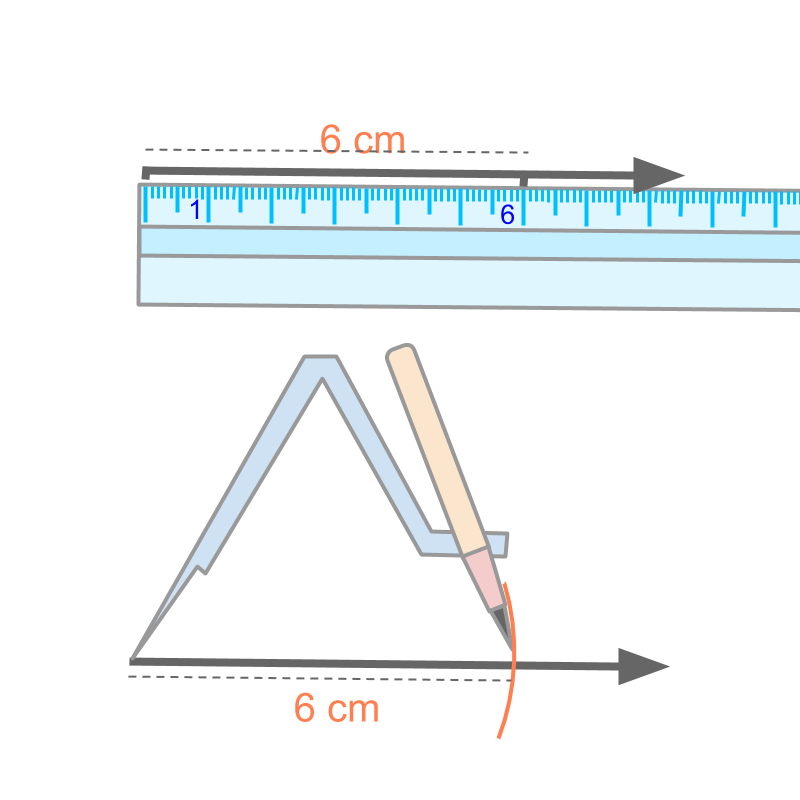Constructing a line Segment: Constructing a line can be achieved by one of the two methods.

• Construct a line and mark the length using a ruler.

• Construct a line and measure the length using a compass to mark an arc on the line.

Constructing a circle and semi-circle

To construct a circle of radius 3$3$cm, a compass is used.?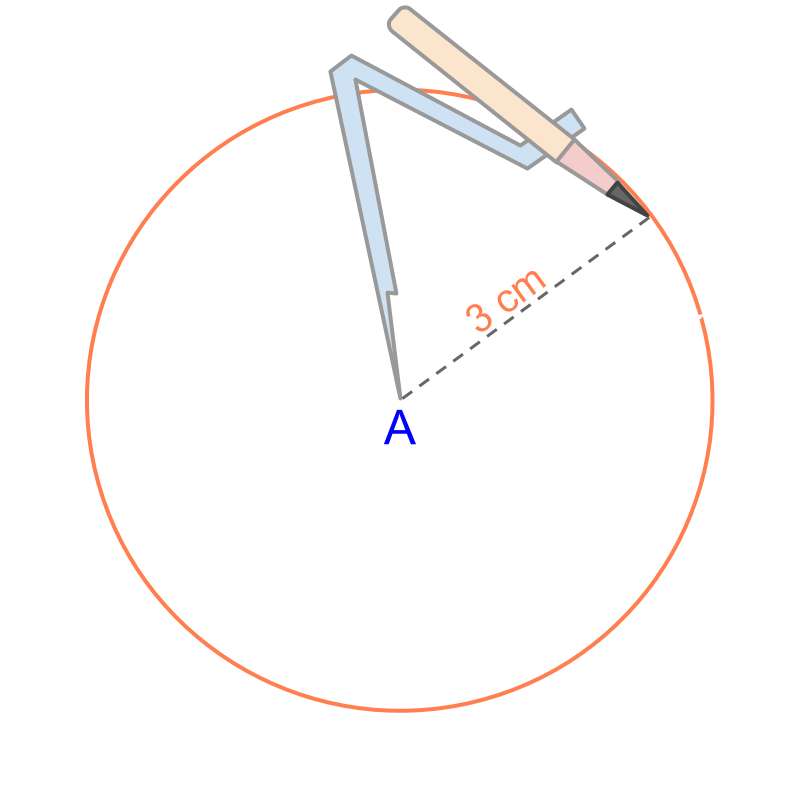Construction of a Circle : Measure the radius in a compass and mark a circle from the center point.

To construct a semi-circle of radius 3$3$cm at point A$A$, a compass is used.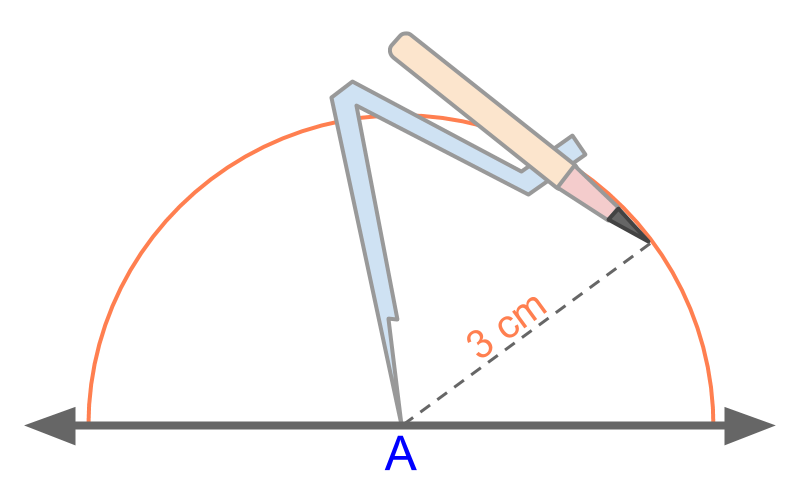Constructing a Semicircle : Measure the radius using a compass and mark the semicircle from the center point.

Construction Line Segment by Copying

A line segment ¯¯¯¯¯¯PQ$\overline{P Q}$ is given. To copy the line segment ¯¯¯¯¯¯PQ$\overline{P Q}$, a compass is used to measure the length of given line segment and mark that in another line.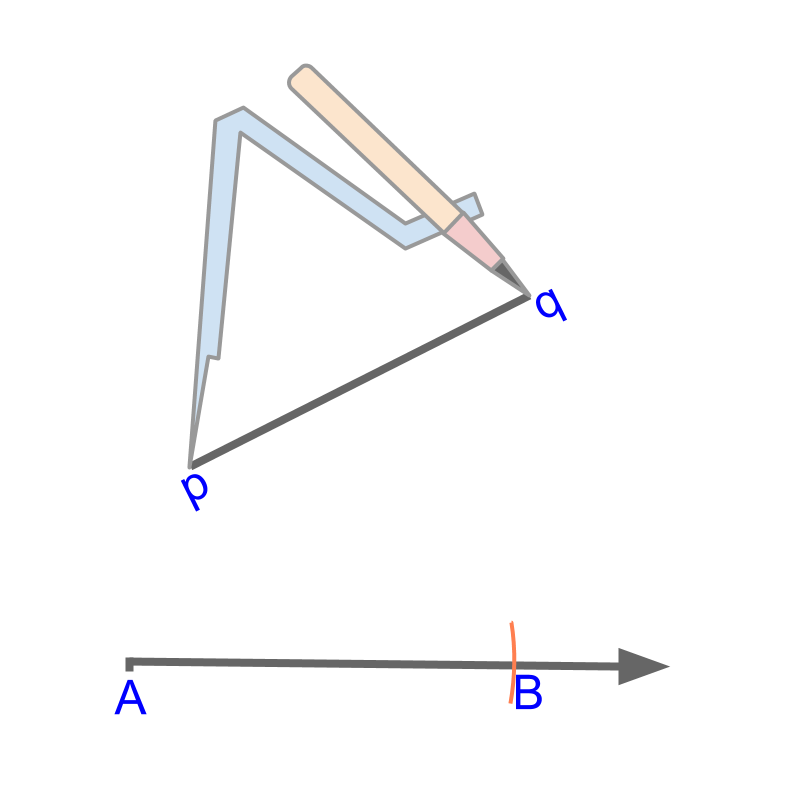Copying a line segment : Copy the length of the given line in a compass and construct an arc on a line.

Copying an angle

The following property helps to copy an angle using compass: "two triangles with identical sides have identical angles".

Given $\angle B A C$. To copy this angle, make a triangle of random sides $\triangle A P Q$. Copy the length of line segment $\overline{A Q}$ to mark point $L$ on the given line. Copy the lengths of line segments $\overline{A P}$ and $\overline{Q P}$ to mark $M$ from points $X$ and $L$ respectively. Join the points $X$ and $M$. The angle $\angle B A C$ is reproduced in $\angle L X M$.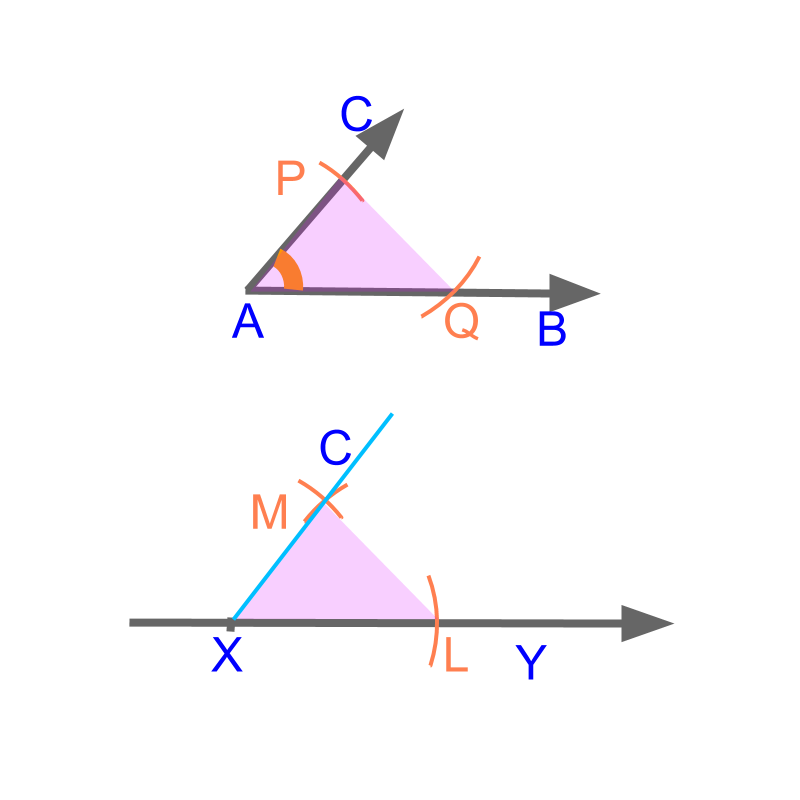Copying a Given Angle : Mark a triangle in the given angle and reproduce the sides of a triangle which copies the angle.

summary

Construction of a line segment : The given length is measured using a ruler / scale and the line segment is marked. Construction of a circle of given radius : The given radius is measured in a compass and the circle is drawn. Construction of a semi circle of given radius : The given radius is measured in a compass and semi circle is drawn. Construction of a line by copying another line : The length of the given line is measured using a compass and the line segment is marked. Construction of an angle by copying another angle : A triangle is marked in the given angle and the sides of the triangle are reproduced to reproduce the angle.

Outline